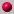# Math 5335: Class Outlines, Fall 201112/14/11: Final Exam. [Text: Sections 9.3-8, Chapter 10]12/14/11: Office hours: 10:30-12:00.12/13/11: Office hours: 11-12:30 and 3:30-5:10 p.m.12/12/11: Review via discussion of Sample Exam III. Solutions of problems which we did not discuss will be posted on the class web page. [Text: Sections 9.3-8, Chapter 10]12/7/11: Homework 10 due and discussed. Lines through a given point perpendicular and parallel to a given line. Area in Poincaré geometry. [Sections 10.3-4]12/5/11: Length, angle measure in Poincaré geometry. Parallelity: ultra and asymptotic. The cross ratio. [Sections 10.1-3 (through Theorem 7)]11/30/11: Homework 9 due and discussed. Congruence axioms (continued). Dedekind's axiom. Parallel axiom. Euclidean geometry is categorical, but is it consistent? [Sections 9.4-8]11/28/11: Betweenness and congruence axioms. [Sections 9.3-4 (through Proposition 12)]11/23/11: Black Wednesday: this pre-Thanksgiving class, along with my office hours this week, is canceled and will be made up through extra office hours before the Final Exam. Happy Thanksgiving!11/21/11: Discussion of Midterm Exam II. [Text: Sections 7.7-8, Chapter 4 through Section 4.8, Chapters 5, 6, 8, and 9 through Section 9.2, skipping the 9-team geometry]11/16/11: Midterm Exam II. [Text: Sections 7.7-8, Chapter 4 through Section 4.8, Chapters 5, 6, 8, and 9 through Section 9.2, skipping the 9-team geometry]11/14/11: Review via discussion of Sample Exam II. [Text: Sections 7.7-8, Chapter 4 through Section 4.8, Chapters 5, 6, 8, and 9 through Section 9.2, skipping the 9-team geometry]11/9/11: Homework 8 due and discussed. Axiomatic geometry. Incidence axioms. Other models of geometry. [Text: Introduction to Chapter 9 and Sections 9.1-2]11/7/11: Conformal mappings: similarity, inversion in a circle, angles between curves. [Text: Sections 8.1-3]11/2/11: Homework 7 due and discussed. Polygons and circles. [Text: Sections 6.2-3]10/31/11: Trigonometry. Trigonometry of triangles. Quadrangles. [Text: Sections 5.1-2 and 6.1]10/26/11: Homework 6 due and discussed. Triangles: the Euler line, angle bisectors and incenter. [Text: Section 4.8]10/24/11: Triangles: orthocenter, area, Heron's formula, circumcenter. [Text: Sections 4.5-7]10/19/11: Homework 5 due and discussed. A few words on classification theorems for isometries. Symmetries of figures. Triangles: angle sum, centroid. [Text: Sections 7.7-8 and 4.1-4]10/17/11: Midterm I discussed. [Text: Chapters 1, 2, skipping Sections 2.1 - 2.2, Chapter 3, Sections 7.1-5]10/12/11: Midterm Exam I. [Text: Chapters 1, 2, skipping Sections 2.1 - 2.2, Chapter 3, Sections 7.1-5]10/5/11: Homework discussion. Isometries: reflections, central inversion, rotations. [Text: Sections 7.3-5]10/3/11: Homework problem 3.8.24. Isometries: identity, translations. [Text: Sections 7.1-2]9/28/11: Homework due at the beginning of class. Homework discussion. Congruence of triangles. Life in barycentric coordinates. Changes of coordinates. [Text: Sections 3.5-7]9/26/11: Barycentric coordinates. Linear transformations and isometries. Congruence. [Text: Sections 3.2-4]9/19/11: Angles and angular measure. Arccos. [Text: Sections 2.1 (through Definition 3), 2.3-4]9/14/11: Homework due at the beginning of class. Homework discussion (Problems 1.7.21, 26, and 28). Lengths for line segments (the triangle inequality, Pythagorean theorem). Distance from a point to a line. [Text: Sections 1.5-6]9/12/11: Subsets of lines: segments and rays. The concept of betweenness. Lengths for line segments (through Cauchy-Schwarz). [Text: Sections 1.3-5 through Lemma 29]9/7/11: Introduction. Points, lines, and incidence. Parametric equations of lines. Some Linear Algebra. A proof of Euclid's axiom. (It is rather a proof of the fact that in our model of geometry, Euclid's axiom is true.) Orthogonal vectors. Normal equations of lines. [Syllabus. Review Linear Systems of Equations. Section 1 of Description of 5335 by Prof. Roberts. Text: Chapter 1 through Section 1.2. Section 1.4 through Lemma 23]